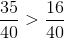MAP 4th Grade Math : Numbers and Operations

Example Questions

Example Question #1 : Numbers And Operations

Select the decimal that is equivalent to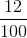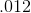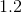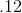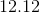Explanation:is twelve hundredths.is twelve hundredths. When we say a decimal, we say the number and add the place-value of the last digit.

Example Question #1 : Numbers And Operations

Fill in the blank with the missing fraction.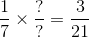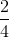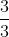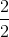Explanation:

In this problem we are making equivalent fractions. In order to make equivalent fractions you have to multiply the numerator and the denominator by the same number.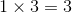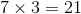Example Question #3 : Numbers And Operations

Select the symbol to correctly fill in the blank below.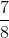__________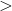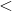Explanation:

To compare fractions, we need to first make common denominators.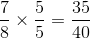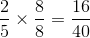Now that we have common denominators, we can compare numerators. The fraction with the bigger numerator has the greater value.